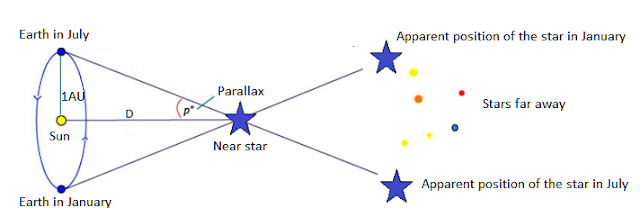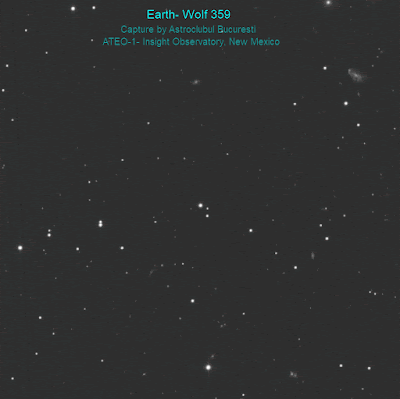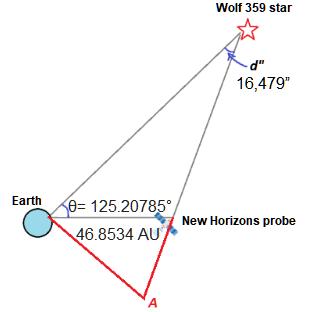-->

# Bringing the Universe to Classrooms and Homes around the World!

## Friday, July 3, 2020

### Wolf 359 Star Parallax

Using Insight Observatory's 16" f/3.75 Dream Aerospace Systems astrograph reflector (ATEO-1) remote telescope, a Romanian team of amateur astronomers participated in the international project #NHParallax. This project is aimed to highlight the parallax effect by comparing image frames from the New Horizons space probe combined with frames obtained by ground-based remote telescope ATEO-1 located in New Mexico, USA.

Introduction:
Historically, the first determination of the distance to the stars was made by astronomer Friedrich Bessel in 1838 for the star 61 Cygni using the parallax method. This method remained the standard procedure for calibrating other methods for determining distances in the Universe. The parallax of a star is the angle through which the radius of the Earth's orbits is seen from the star when it is perpendicular to the Earth-Star direction. The simplified model of the parallax effect is illustrated in Figure 1. It involves a change in the apparent position of a star relative to the stars from the background when observed from two different locations. This is quantified by the angle p - called the parallax.Figure 1 - Parallax Effect

For the calculation of the distance, we refer to Figure 1.

The following equations can be written:

tg (p ”) = Earth-Sun Distance / Sun-Star Distance
tg (p ”) = 1UA / D, from which it follows that:
D = 1 / tg (p ”)
Since the angle p ”is very small (less than one second of arc) we can approximate tg (p”) = p ”and the formula becomes:
D = 1 / p ”,
where D is expressed in parsecs (pc)
p” is expressed in arcseconds and 1 AU represents an astronomical unit, ie the average Earth-Sun distance.

Traditionally the distances to Proxima Centauri and Wolf 359 were calculated using this method and resulted in the following data confirmed by the specialized space missions Hiparchos and Gaia:

p”Wolf 359 = 0.41516” ± 0.000162 ”

The distance to the star Wolf 359 = 2,409 ± 0.009 pc, ie 7.86 light-years (+/-0.03 ly)

Remarks:
On April 22, 2020, we made observations with the ATEO-1 remote telescope in tandem with the New Horizons space probe at nearby star Wolf 359. The advantage of these tandem observations is that the New Horizons probe is 46 times the Earth-Sun distance, which causes the perspective of the probe on the star Wolf 359 to change visibly from the perspective observed on earth. With such a large distance between the two observation points (Earth - New Horizons), the parallax effect is easy to highlight by comparing photos taken from Earth with those received from the New Horizons probe. Here is the animation which shows a stereo view of this phenomenon.Wolf 359 Star animation with image data acquired from the New Horizons space probe  and Insight Observatory's ATEO-1 remote telescope located in New Mexico.

The raw images obtained by the New Horizons space probe were downloaded from the website below dedicated to this project:

http://pluto.jhuapl.edu/Learn/Parallax/Parallax-Images.php

The camera used by the space mission was the Long-Range Reconnaissance Imager (LORRI). The raw images required some processing to eliminate image artifacts. This was done in the following steps:

- Creation of synthetic flat and removal of cosmic rays and hot pixels.
- Slight convolution of stars.

In order to measure the parallax angle, we must calibrate the two images in coordinates relative to the celestial sphere. This calculation was performed using the Astrometry.net program.

We determined the astrometric solution of the plate in the ICRS J2000 (International Celestial Reference System). Because the LORRI camera aboard the New Horizons spacecraft was designed to take images of trans-Neptunian objects, its resolution is only 4,09 arcsec/pixel as opposed to the much better resolution ATEO-1 telescope provides, ie 1,237 arcsec /pixel.

Results:
Since the geometry of the observations is more general than in the simplified model, respectively the Wolf 359 star is not centered in relation to the observation base. We first measured the angular distance between the star and the New Horizons probe at the date of the observation. The following were obtained:

θNH-Wolf359 = 125.20785° - The angular separation between New Horizons and Wolf 359.

According to the Jet Propulsion Laboratory website at the time of the observations, the distance between the Earth and the New Horizons spacecraft was 46.8534 AU, ie 7.028 billion kilometers. We measured the position of the Wolf star on the New Horizons frames and on the images obtained with the ATEO-1 telescope. We used all the images (3 provided by the New Horizons probe and 6 purchased with the telescopes we used) and averaged these measurements. To evaluate the measurement error we calculated their standard deviation. The results obtained are presented in Table 1.Table 1: Wolf 359 star coordinates in both images obtained by the  New Horizons probe and ATEO-1 telescope - ICRS2000.

An important aspect of these measurements, given that the pixel size is large compared to the star's profile, is the algorithm for identifying the centroid of each star in the images. For this, we used two methods: the position of the brightest pixel and the algorithm proposed by the AstroImageJ program.

To calculate the parallax, we used the formula for calculating the angular separation (Jean Meus - Astronomical Algorithms). In this formula, α and δ are the right ascension and the declination and the indices 1 and 2 correspond to the measurements with our telescope, respectively with the New Horizons probe.

cos d = sinδ1 sinδ2 + cosδ1 cosδ2 cos (α1 - α2)

Based on this formula we obtained the following parallaxes. I marked it with d to differentiate it from the meaning described in the introduction.

d”Wolf359 = 16,479” ± 3,390 ”Figure 3: The geometry of the Earth, New Horizons space probe, and Wolf 359 star on April 22, 2020.

Taking into account the geometry of the observation (Figure 3) the distance can be calculated using the formula:

distEarth-Wolf359 ≈ distEarth-New Horizon / tg (d”) * sin (θ),

where distEarth-Wolf359 is the distance from Earth to the Wolf star, distEarth-New Horizon= 46.8534 AU is the distance from Earth to the New Horizons probe, and sin (θ) is the factor that takes into account the geometry of the observation.

We obtained the following distance for Wolf star:

distEarth-Wolf359 = 7,576 ± 1,559 light-years.

Conclusions:
This result corresponds to the recent determinations reported by the Gaia space mission and presented in the introduction. Our measurement has a lower degree of accuracy due to the low resolution of the LORRI (Long Range Reconnaissance Imager) camera with which the images from the New Horizons probe were recorded. This camera has a resolution of 4.09 ”/ pixel which means that a one-pixel position measurement error is a 10-20% parallax error (depending on the star). The approximations made in this calculation are insignificant in relation to the error in determining the position.

NASA's #NHParallax project to measure parallax by performing tandem observations on Wolf 359 and Proxima Centauri stars with the New Horizons spacecraft was purely educational, with NASA encouraging amateur astronomers around the world to make observations with their instruments at the same time as the space probe.

More details about this project can be found on the official website of the New Horizons mission:

http://pluto.jhuapl.edu/Learn/Get-Involved.php#NHparallax

The FITS files from the New Horizon space probe can be downloaded here:

http://pluto.jhuapl.edu/Learn/Parallax/Parallax-Images.php

The movement of the two stars is very difficult to visualize in the frames recorded on Earth, six months away because the parallax angle is extremely small compared to the star profile caused of the atmospheric disturbance recorded by the best observatories. The present project has managed to clearly illustrate this effect.

Authors Affiliation:
Daniel Bertesteanu - Bucharest Astroclub
Marcel Popescu - Astronomical Institute of the Romanian Academy
Marian Naiman - Bucharest Astroclub